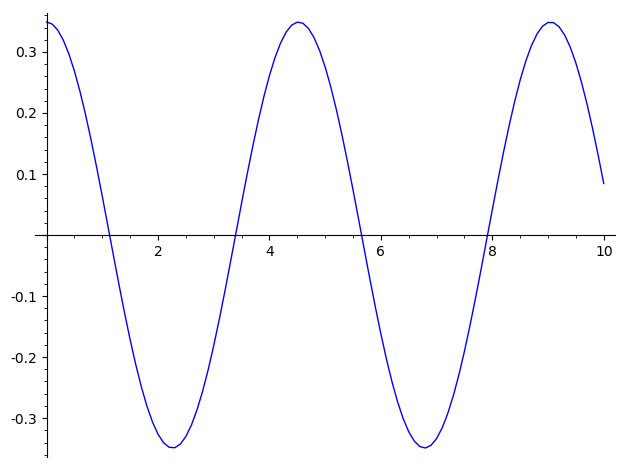# type error solving system of differential equations numerically

I tried to solve a differential system numerically as follows:

R = 5
g = 9.8
t = var('t')
x = function('x')(t)
y = function('y')(t)
de1 = diff(x,t) - y == 0
de2 = diff(y,t) + (g/R)*sin(x) == 0
desolve_system_rk4([de1,de2],[x,y],ics = [0,(20/180)*pi,0],ivar=t)


and got a type error:

Traceback (most recent call last)
...
TypeError: Error executing code in Maxima
CODE:
sage4 : rk(['diff('x(_SAGE_VAR_t),_SAGE_VAR_t,1)-'y(_SAGE_VAR_t)=0,'diff('y(_SAGE_VAR_t),_SAGE_VAR_t,1)+1.96*sin('x(_SAGE_VAR_t))=0],['x(_SAGE_VAR_t),'y(_SAGE_VAR_t)],[%pi/9,0],[_SAGE_VAR_t,0,10,0.1])        \$
Maxima ERROR:

rk: variable name expected; found: 'x(_SAGE_VAR_t)
-- an error. To debug this try: debugmode(true);

edit retag close merge delete

Sort by » oldest newest most votedThat is not the type of input that desolve_system_rk4 accepts. As written in the documentation and seen in the example, it accepts a list of right-hand sides of first-order differential equations, like this:

R = 5
g = 9.8
var('x,y,t')
de1 = y
de2 = -(g/R)*sin(x)
sol_points = desolve_system_rk4([de1,de2],[x,y],ics = [0,(20/180)*RR.pi(),0],ivar=t)
sol_points_xy = [(x,y) for (x,y,_) in sol_points]
list_plot(sol_points_xy, plotjoined=True)more

1